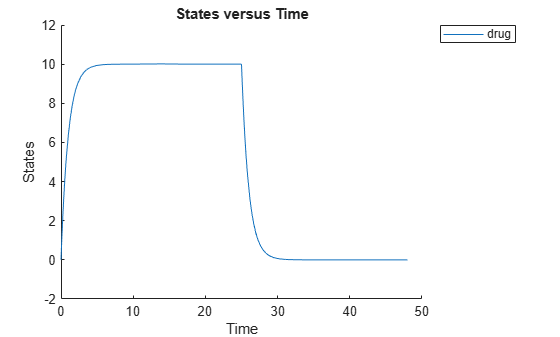## Syntax

```doseObj2 = adddose(modelObj, 'DoseName') doseObj2 = adddose(modelObj, 'DoseName', 'DoseType') doseObj2 = adddose(modelObj, doseObj) ```

## Arguments

 `modelObj` `Model object` to which you add a dose object. `DoseName` Name of a dose object to construct and add to a model object. `DoseName` is the value of the dose object property `Name`. `DoseType` Type of dose object to construct and add to a model object. Enter either `'schedule'` or `'repeat'`. `doseObj` Dose object to add to a model object. Created with the constructor `sbiodose`.

## Outputs

 `doseObj2` `ScheduleDose object` or `RepeatDose object`. A `RepeatDose` or `ScheduleDose` object defines an increase (dose) to a species amount during a simulation.

## Description

Before using a dose object in a simulation, use the `adddose` method to add the dose object to a SimBiology® ```model object```. Then, set the `Active` dose object property to `true`.

```doseObj2 = adddose(modelObj, 'DoseName')``` constructs a SimBiology RepeatDose object (`doseObj2`), assigns `DoseName` to the property `Name`, adds the dose object to a SimBiology model object (`modelObj`), and assigns `modelObj` to the property `Parent`.

```doseObj2 = adddose(modelObj, 'DoseName', 'DoseType')``` constructs either a SimBiology `ScheduleDose` object or `RepeatDose` object (`doseObj`).

```doseObj2 = adddose(modelObj, doseObj)``` adds a SimBiology dose object (`doseObj`) to a SimBiology model object `(modelObj)`, copies the dose object to a second dose object (`doseObj2`), and assigns `modelObj` to the property `Parent`. The `Active` property of `doseObj2` is set to `false` by default.

Note

Alternatively, you can create a dose object using `sbiodose` as a standalone dose object, which you can apply to different models. For details, see Creating Doses Programmatically.

## Examples

collapse all

This example shows how to add a constant-rate infusion dose to a one-compartment model.

Background

Suppose you have a one-compartment model with a species named `drug` that represents the total amount of drug in the body. The drug is removed from the body via the first-order elimination represented by the reaction `drug -> null`, with the elimination rate constant `ke`. In other words, the drug concentration versus the time profile follows the monoexponential decline ${C}_{t}={C}_{0}{e}^{-{k}_{e}t}$, where ${C}_{t}$ is the drug concentration at time t, ${C}_{0}$ is the initial concentration, and `ke` is the elimination rate constant. This example shows how to set up such a one-compartment model and add an infusion dose at a constant rate of 10 mg/hour for the total dose amount of 250 mg.

Create a One-Compartment Model

Create a SimBiology model named `onecomp`.

`m1 = sbiomodel('onecomp');`

Define the elimination of the drug from the system by adding a reaction `drug -> null` to the model.

`r1 = addreaction(m1,'drug -> null');`

The species `drug` is automatically created and added to the compartment. The `null` species is a reserved species that acts as a sink in this reaction.

Add a mass action kinetic law to the reaction. This kinetic law defines the drug elimination to follow the first-order kinetics.

`k1 = addkineticlaw(r1,'MassAction');`

Define the elimination rate parameter `ke` and add it to the kinetic law.

`p1 = addparameter(k1,'ke','Value',1.0,'ValueUnits','1/hour');`

Specify the rate parameter `ke` as the forward rate parameter of the reaction by setting the `ParameterVariableNames` property of kinetic law object `k1`. This allows SimBiology to determine the reaction rate for `drug -> null` reaction.

`k1.ParameterVariableNames = 'ke';`

Set Up an Infusion Dose

Add a dose object to the model using the `adddose` method. Specify the amount of the dose (`Amount`), the dose target (`TargetName`), and the infusion rate (`Rate`). You also need to set the `Active` property of the dose object to `true` so that the dose is applied to the model during simulation.

```d1 = adddose(m1,'InfusionDose'); d1.Amount = 250; d1.TargetName = 'drug'; d1.Rate = 10; d1.RateUnits = 'milligram/hour'; d1.Active = true;```

Simulate the Model

Change the simulation stop time to 48 hours to see the complete time course. Set the compartment unit to liter.

```cs = getconfigset(m1); cs.StopTime = 48; cs.TimeUnits = 'hour'; c1 = m1.Compartments; c1.Units = "liter"; sd = sbiosimulate(m1);```

Plot Results

Plot the concentration versus the time profile of the drug in the system.

`sbioplot(sd);`## Version History

Introduced in R2010a

expand all